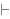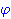New Foundations Explorer < Previous   Next > Nearby theorems Mirrors  >  Home  >  NFE Home  >  Th. List  >  idALT Unicode version

Theorem idALT 20
 Description: Principle of identity. Theorem *2.08 of [WhiteheadRussell] p. 101. This version is proved directly from the axioms for demonstration purposes. This proof is a popular example in the literature and is identical, step for step, to the proofs of Theorem 1 of [Margaris] p. 51, Example 2.7(a) of [Hamilton] p. 31, Lemma 10.3 of [BellMachover] p. 36, and Lemma 1.8 of [Mendelson] p. 36. It is also "Our first proof" in Hirst and Hirst's A Primer for Logic and Proof p. 17 (PDF p. 23) at http://www.mathsci.appstate.edu/~hirstjl/primer/hirst.pdf. For a shorter version of the proof that takes advantage of previously proved theorems, see id 19. (Contributed by NM, 5-Aug-1993.) (Proof modification is discouraged.) Use id 19 instead. (New usage is discouraged.)
Assertion
Ref Expression
idALTProof of Theorem idALT
StepHypRef Expression
1 ax-1 5 . 22 ax-1 5 . . 33 ax-2 6 . . 342, 3ax-mp 8 . 251, 4ax-mp 8 1Colors of variables: wff setvar class Syntax hints:wi 4 This theorem was proved from axioms:  ax-1 5  ax-2 6  ax-mp 8 This theorem is referenced by: (None)
 Copyright terms: Public domain W3C validator